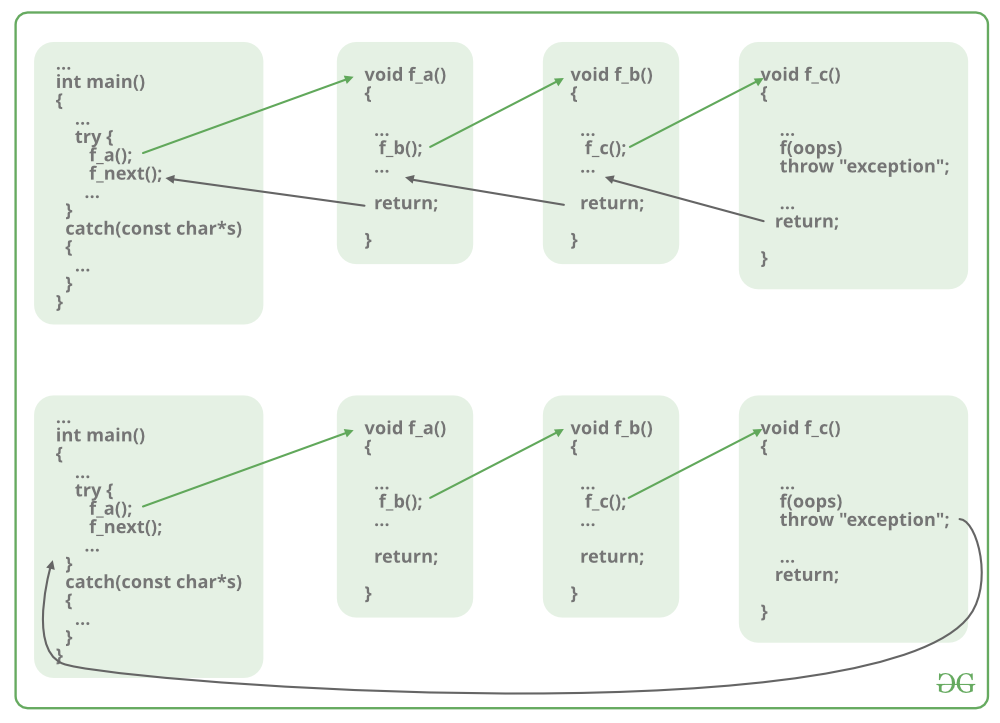Open in App
Not now

# return statement in C++ with Examples

• Difficulty Level : Basic
• Last Updated : 04 Jan, 2023

Pre-requisite: Functions in C++

The return statement returns the flow of the execution to the function from where it is called. This statement does not mandatorily need any conditional statements. As soon as the statement is executed, the flow of the program stops immediately and returns the control from where it was called. The return statement may or may not return anything for a void function, but for a non-void function, a return value must be returned.

Syntax:

`return[expression];`There are various ways to use return statements. A few are mentioned below:

### 1. Methods not returning a value

In C++ one cannot skip the return statement when the methods are of the return type. The return statement can be skipped only for void types.

#### Not using a return statement in void return type function

When a function does not return anything, the void return type is used. So if there is a void return type in the function definition, then there will be no return statement inside that function (generally).

Syntax:

```void func()
{
.
.
.
}```

Example:

## C++

 `// C++ code to show not using return``// statement in void return type function``#include ``using` `namespace` `std;`` ` `// void method``void` `Print()``{``    ``cout << ``"Welcome to GeeksforGeeks"``;``}`` ` `// Driver method``int` `main()``{`` ` `    ``// Calling print``    ``Print();`` ` `    ``return` `0;``}`

Output

`Welcome to GeeksforGeeks`

#### Using the return statement in void return type function

Now the question arises, what if there is a return statement inside a void return type function? Since we know that, if there is a void return type in the function definition, then there will be no return statement inside that function. But if there is a return statement inside it, then also there will be no problem if the syntax of it will be:

Syntax:

```void func()
{
return;
}```

This syntax is used in function just as a jump statement in order to break the flow of the function and jump out of it. One can think of it as an alternative to “break statement” to use in functions.

Example:

## C++

 `// C++ code to show using return``// statement in void return type function``#include ``using` `namespace` `std;`` ` `// void method``void` `Print()``{``    ``cout << ``"Welcome to GeekforGeeks"``;`` ` `    ``// void method using the return statement``    ``return``;``}`` ` `// Driver method``int` `main()``{`` ` `    ``// Calling print``    ``Print();``    ``return` `0;``}`

Output

`Welcome to GeekforGeeks`

But if the return statement tries to return a value in a void return type function, that will lead to errors.

Incorrect Syntax:

```void func()
{
return value;
}```

Example:

## C++

 `// C++ code to show using return statement``// with a value in void return type function``#include ``using` `namespace` `std;`` ` `// void method``void` `Print()``{``    ``cout << ``"Welcome to GeeksforGeeks"``;`` ` `    ``// void method using the return``    ``// statement to return a value``    ``return` `10;``}`` ` `// Driver method``int` `main()``{`` ` `    ``// Calling print``    ``Print();`` ` `    ``return` `0;``}`

Output:

`warning: 'return' with a value, in function returning void`

### 2. Methods returning a value:

For methods that define a return type, the return statement must be immediately followed by the return value of that specified return type.

Syntax:

```return-type func()
{
return value;
}```

Example:

## C++

 `// C++ code to illustrate Methods returning``// a value using return statement``#include ``using` `namespace` `std;`` ` `// non-void return type``// function to calculate sum``int` `SUM(``int` `a, ``int` `b)``{``    ``int` `s1 = a + b;`` ` `    ``// method using the return``    ``// statement to return a value``    ``return` `s1;``}`` ` `// Driver method``int` `main()``{``    ``int` `num1 = 10;``    ``int` `num2 = 10;``    ``int` `sum_of = SUM(num1, num2);``    ``cout << ``"The sum is "` `<< sum_of;``    ``return` `0;``}`

Output:

`The sum is 20`

Note: We can only return a single value from a function using return statement. To return multiple values, we can use pointers, structures, classes, etc. To know more, click here.

My Personal Notes arrow_drop_up